Transport phenomena (diffusion, thermal conductivity, viscosity)

In nonequilibrium systems, special irreversible processes arise, called transfer phenomena , which result in the spatial transfer of mass, energy, and momentum.

Diffusion is due to mass transfer, thermal conductivity due to energy transfer, and viscosity due to momentum transfer .

To characterize irreversible transport processes, the parameters of the thermal motion of molecules are introduced: the average number of collisions of a molecule per unit timeand average mean free path of molecules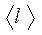.

Average number of collisions of a molecule in 1 s :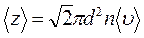,

where d is the effective diameter of the molecules, i.e. the minimum distance that the centers of two molecules come closer in a collision,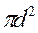- effective cross section of molecules,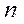- concentration of molecules,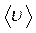- the arithmetic average speed of the molecules.

The mean free path of molecules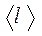, i.e. The average path traveled by a molecule between two successive collisions:.

When considering one-dimensional transport phenomena, the reference frame is chosen so that the x axis is oriented in the direction of transport.

1. Diffusion. The diffusion phenomenon consists in the fact that spontaneous interpenetration and mixing of particles of two contacting gases, liquids, and even solids occurs. Diffusion reduces to mass transfer, arises and continues until the density gradient is nonzero at the interface between the two media.

Density gradientalong the selected x axis, perpendicular to the plane of contact of the two media, is denoted asand shows how fast the density changesfrom point to point along the x axis .

Quantitatively, the phenomenon of diffusion obeys Fick's law :,

Where- mass flow density, that is, a value determined by the mass of gas diffusing through a unit area S per unit time,- the gas density gradient in the x direction perpendicular to the selected site S ,

D is the diffusion coefficient .

The minus sign in the above formula means that mass transfer occurs in the direction of decreasing density.

According to the molecular-kinetic theory of an ideal gas, the diffusion coefficient D :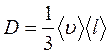,

Where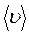Is the average thermal velocity of the molecules,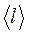- the average free path of the molecules.

2. Thermal conductivity. If the temperature in one region of the gas is higher than in the other, then over time, due to constant collisions of molecules, the process of equalization of the average kinetic energies of the molecules occurs, that is, the process of temperature equalization. This process of energy transfer, called thermal conductivity , occurs and continues until the temperature gradient is non-zero at the interface between the two parts of the gas.The temperature gradient T of the gas along the selected x axis, perpendicular to the plane of contact of the two parts of the gas having different temperatures, is denoted as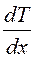and shows how quickly the gas temperature changes from point to point along the x axis .

Quantitatively, thermal conductivity obeys the Fourier law :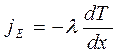,

Where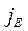- the heat flux density , determined by the energy transferred in the form of heat through a unit area S per unit time,- the temperature gradient in the x direction perpendicular to the selected site S ,- coefficient of thermal conductivity .

The minus sign in the above formula means that during thermal conductivity, energy is transferred in the direction of decreasing temperature.

According to the molecular-kinetic theory of an ideal gas, the coefficient of thermal conductivity:,

Where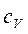- specific heat of the gas during the isochoric process (the amount of heat required for isochoric heating of 1 kg of gas per 1 K ),- gas density,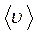Is the average thermal velocity of the molecules,- the average free path of the molecules.

3. Viscosity . Viscosity is a property of a liquid or gas due to internal friction between adjacent parallel layers of liquid or gas moving at different speeds. As a result, the momentum of the layer moving faster decreases, and the momentum of the moving slower increases, which slows down the layer moving faster and accelerates the layer moving slower. In other words, internal friction leads to the transfer of momentum from one moving layer of a liquid or gas to another layer in contact with it.

Quantitatively, the force of internal friction between two adjoining layers of a liquid or gas obeys Newton's law :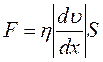,

where h is the coefficient of dynamic viscosity ,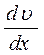- a velocity gradient showing the rate of change of the flow velocity of a liquid or gas from layer to layer in the x direction perpendicular to the direction of movement of the layers,

S is the contact area of ​​the liquid or gas layers affected by the internal friction force F.

Newton's law for internal friction can be represented as:,

Where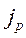- pulse flux density - a value determined by the pulse transferred per unit time through a unit area S of the contact of the liquid or gas layers in the x- axis direction perpendicular to the direction of movement of the liquid or gas layers.

The minus sign in the above formula means that the impulse is transferred from layer to layer of the liquid (gas) in the direction of decreasing speed of their movement.

According to the molecular-kinetic theory of an ideal gas, the dynamic viscosity coefficient of an ideal gas h is determined as follows:,

Where- gas density,Is the average thermal velocity of the molecules,- the average free path of the molecules.

; Date Added: 2014-02-02 ; ; views: 54314 ; Does published material infringe copyright? | | Personal Data Protection | ORDER JOB

Did not find what you were looking for? Use the search:

Best sayings: Learn to study, not learning! 10241 -| 7810 -or read everything ...